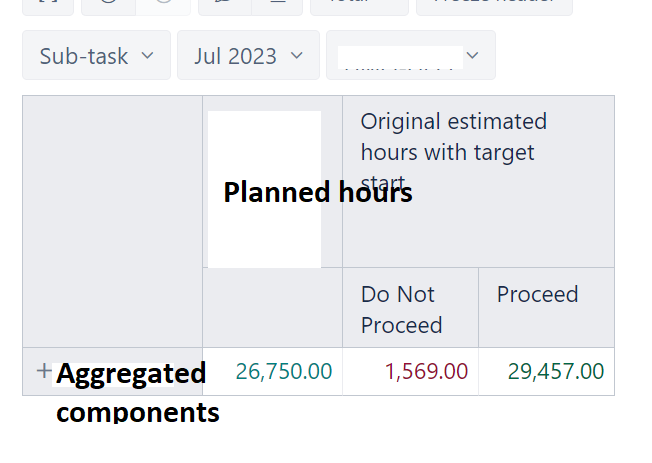# Sum Original estimate hours

Hello,

We would like to add a calculation below

Planned hours [custom field] - Original Estimated hours if decision is “Proceed”

Proceed and Do Not Proceed is a custom fieldTried this formula but not working as expected.

[Measures].[Planned Capacity with target start]-[Measures].[Original estimated hours with target start]

Hi,

Have you tried the following formula for the calculation using the tuple?

``````[Measures].[Planned Capacity with target start]
-
(
[Measures].[Original estimated hours with target start],
[Decision].[Proceed]
)
``````

The report should not contain the Decision dimension for this formula to work.

Kindly,
Janis, eazyBI support

1 Like

Thanks @janis.plume . This works